Effect of interfacial coupling on rectification in organic spin rectifiers
Hu Gui-Chao†, Zuo Meng-Ying, Li Ying, Zhang Zhao, Ren Jun-Feng, Wang Chuan-Kui
College of Physics and Electronics, Shandong Normal University, Jinan 250014, China

Corresponding author. E-mail: hgc@sdnu.edu.cn

*Project supported by the National Natural Science Foundation of China (Grant No. 1374195), the Natural Science Foundation of Shandong Province, China (Grant No. ZR2014AM017), and the Excellent Young Scholars Research Fund of Shandong Normal University, China.

Abstract

The effect of interfacial coupling on rectification in an organic co-oligomer spin diode is investigated theoretically by considering spin-independent and spin-resolved couplings respectively. In the case of spin-independent coupling, an optimal interfacial coupling strength with a significant enhanced rectification ratio is found, whose value depends on the structural asymmetry of the molecule. In the case of spin-resolved coupling, we found that only the variation of the interfacial coupling with specific spin is effective to modulate the rectification, which is due to the spin-filtering property of the central asymmetric magnetic molecule. A transition of the spin-current rectification between parallel spin-current rectification and antiparallel spin-current rectification may be observed with the variation of the spin-resolved interfacial coupling. The interfacial effect on rectification is further analyzed from the spin-dependent transmission spectrum at different biases.

PACS: 73.63.–b; 75.10.Lp; 85.75.–d
Keyword: organic spintronics; organic ferromagnet; rectification; interfacial coupling
1. Introduction

Organic spintronics has attracted more and more attention in recent years for the promise of developing cheap and low-weight devices. The weak spin– orbital interaction and hyperfine interaction in organic materials are advantageous for spin transport, which opens a new arena to design excellent organic spin functional devices. In this field, organic ferromagnets are particularly intriguing since they combine the advantages of organic materials and ferromagnets. Organic ferromagnets are usually synthesized artificially due to the absence of d electrons. So far, a series of organic ferromagnets have been synthesized and measured, such as poly(1, 4-bis-(2, 2, 6, 6-tetramethyl-4-oxy-4-piperidyl-1-oxyl)-butAdiin) (poly-BIPO), [5, 6] m-polydiphenylcarbene (m-PDPC),  pyrolysed polyacrylonitrile (pyro-PAN),  V[TCNE]x (TCNE = tetracyanoethylene), [9, 10] and 2-benzimidazolyl nitronyl nitroxide (2-BIMNN). Triggered by the great progress in molecular electronics in recent years, the design of organic spintronic devices based on organic ferromagnets has become attractive and shown promise in organic spintronic device design, such as spin valves, [12, 13] spin filters, [14, 15] and spin diodes.

Spin diodes are especially fascinating because of their important role in logical circuits. As is well known, the charge current is a scalar which is the sum of the currents with different spins. However, the spin current is a vector. With the definition of difference between spin-up and spin-down currents, [16, 17] it contains two characteristics: the amplitude and the spin-polarized orientation of the spin current. Thus spin-current rectification has two forms: asymmetric amplitude and different spin-polarized orientation of the spin current with the reversal of the bias, which can be called “ parallel spin-current rectification” and “ antiparallel spin-current rectification, ” respectively. Unlike normal charge-current diodes, spin-current rectification requires the introduction of an asymmetric device structure in the spin degree of freedom. In the past decade, the possibility of spin diode behavior has been discussed and measured in a quantum wire, a carbon-nanotube quantum dot, and a semimagnetic semiconductor. The first design with organic molecules was proposed by Dalgleish and Kirczenow. Their ab-initio calculation revealed that a spin-current rectification can be realized by coupling a molecular wire with one ferromagnetic electrode and one nonmagnetic electrode, where the structural asymmetry stems from the external electrodes.

Recently, we also designed a new intrinsic organic spin diode theoretically by using an asymmetric magnetic co-oligomer sandwiched between two identical electrodes, where the built-in asymmetry originates from the central molecule.[17, 18] The model calculations demonstrated that the charge-current rectification and the spin-current rectification may be realized either simultaneously or respectively. The spin rectification can be further modulated by the molecular spin states or the proportion of the co-oligomer.[18, 24] Such a model design has been verified by first-principles calculations with biradical and triradical magnetic molecules.

It is known that the interfacial coupling in organic devices plays a crucial role for their conductivity. For example, the current through the device is sensitive to the interfacial coupling strength, which can be modulated by changing the molecule– electrode distance or the terminal anchoring group.[26, 27] Asymmetric interfacial coupling may also induce molecular rectification.[28, 29] In the case of magnetic junctions, the interfacial coupling may be further spin-dependent due to the spin nondegeneracy of electrodes or molecules, where the interfacial spin polarization as well as the spin-polarized transport can be dramatically modified by the interface.[14, 30] Thus, the interfacial manipulation provides a potential way to modulate the spin-dependent transport in an organic device, which even gives rise to the field of “ spinterfaces.”  For an organic spin rectifier, the interfacial coupling effect needs to be further explored together with the asymmetric bias effect on the device, which has never been considered in previous studies.

In this paper, we theoretically investigate the interfacial coupling effect on the asymmetric spin-dependent transport through the magnetic co-oligomer spin diode, where the interfacial effect will be explored in two aspects: spin-independent coupling and spin-resolved coupling. Both the charge-current rectification and the spin-current rectification of the device will be studied. The paper is organized as follows. Section 2 introduces the adopted model and the calculation method. Section 3 discusses the calculated results and a summary is given in Section 4.

2. Model and method

A quasi-one-dimensional chain is used to model the device. The structure is shown in Fig.  1. An asymmetric organic magnetic co-oligomer is sandwiched between two semi-infinite metallic chains. The central molecule is composed of the left organic ferromagnetic part (poly-BIPO) and the right nonmagnetic part (polyacetylene). Radicals hang on the main chain of the left ferromagnetic part and each contains an unpaired electron. The residual spins of the radicals have a ferromagnetic order at the ground state. The molecule can be described by the Su– Schieffer– Heeger (SSH) model combined with a Kondo term[32, 33]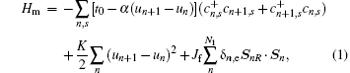where t0 is the hopping integral of π electrons between the n-th and (n+ 1)-th sites in the uniform chain; α is the electron-lattice coupling constant, un is the lattice displacement at site n,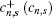is the creation (annihilation) operator of a π electron at the n-th site with spin s; and K is the elastic coefficient of the main chain. The last term describes the spin correlation between the π -electron spin Sn and the residual spin SnR of the radical R with coupling strength Jf; δ n, e means that the side radicals are connected only to the even numbered sites of the left magnetic segment with a total of N1 sites. Under the mean-field approximation, the radical spin SnR is further treated as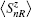, whereis the average value of the spin along the z axis.

 Figure Option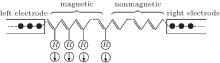Fig.  1. Schematic structure of a metal/magnetic-nonmagnetic co-oligomer/metal spin diode.

The two identical electrodes are described with a simple tight-binding model with on-site energy zero and the nearest hopping integral tf. The interfacial coupling between the electrodes and the molecule is assumed to take place only at the nearest connecting atoms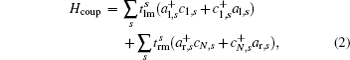where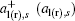denotes the creation (annihilation) operator of an electron with spin s at the terminal atom coupling with the molecule; and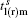is the spin-resolved interfacial hopping integral, which can be further expressed as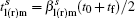. Here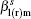is a binding parameter to indicate the coupling strength, and N is the total number of sites in the central molecule.

Applying a bias V, an electric field E is assumed to be generated along the molecule with E = V/[(N − 1)a]. This assumption is reasonable when the applied bias is not too large. The Hamiltonian of the external field can be written as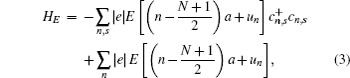with e being the electronic charge and a the lattice constant. The two terms denote the electric potential energies of π electrons and lattice ions, respectively.

Given a bias, the lattice configuration and the electronic structure of the magnetic molecule can be obtained by solving the electronic eigen equation and minimizing the total energy of the molecule within a self-consistent method.[32, 33] Then the spin-dependent current through the device can be calculated by the Landauer– Bü ttiker formula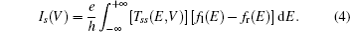Here Tss(E, V) is the spin-dependent transmission coefficient, which is determined from the lattice Green function Gss(E, V) through the Fish– Lee relation as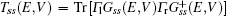, where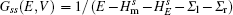with l(r) being the self-energy operator due to the coupling to the left (right) electrode, and Γ l(r) is the broadening matrix. The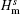and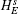correspond to the electron Hamiltonian with spin s in Eqs.  (1) and (3), and fl(r)(E) = 1/{1 + exp[(Eμ l(r))/KBT]} is the Fermi distribution function of the left (right) electrode, where μ l(r) = EF ± eV/2 is the chemical potential and EF is the Fermi energy. The charge current through the device can be obtained as the sum of spin-up and spin-down currents, i.e., Ic = I + I, while the spin current is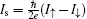.

In the numerical calculation, the parameters are chosen as follows: t0 = 2.5  eV, α = 4.1  eV/Å , K = 21.0  eV/Å 2, J = Jf/t0 = 1.5, and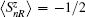, which may describe the co-oligomer of organic ferromagnet poly-BIPO and polyacetylene properly.[32, 33, 35] The lengths of the two segments are set equal with N1 = N2 = 16. The hopping integral in the electrodes is tf = 2.5  eV.

3. Results and discussion

In a real molecular device, the interfacial coupling is affected by several factors, such as the energy level alignment between the molecule and the electrode, anchoring groups, microscopic contact geometries, and even the bias voltage, [26, 36, 37] where a detailed description is beyond a simple tight-binding model. However, physically speaking, two aspects of the interface are important to modulate the transport property, that is, the interfacial coupling strength and the spin-resolved coupling. In the following, we will focus on these two points respectively to study the interfacial effect.

3.1. Interfacial coupling strength

For simplicity, we first consider a symmetric spin-independent interfacial coupling by setting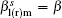. It is well known that in a symmetric molecular device, the interfacial coupling strength modulates the interfacial tunneling barrier, and then changes the device conductance exponentially. However, for a co-oligomer diode, such interfacial modulation is expected to exist under both negative and positive biases. Thus, the rectification comes out as their competition and cannot be deduced directly.

In Fig.  2, we show the calculated charge current and spin current through the organic spin rectifier with three different interfacial coupling strengths, β = 0.2, 0.6, and 1.2. Apparently, both the charge current and the spin current manifest rectification in all three cases, where the current in the positive region is larger than that in the negative bias region. Here the spin-current rectification appears in the form of parallel spin-current rectification,  where only the magnitude of the spin current is asymmetric while its spin-polarized orientation keeps unchanged. With β increasing from 0.2 to 1.2, a significant enhancement of the current is observed in the positive bias region, but the increase in the negative bias region is relatively small.

 Figure Option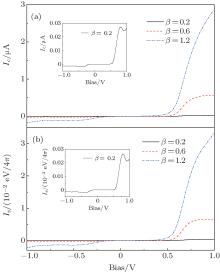Fig.  2. (a) Charge current and (b) spin current as a function of bias voltage with different interfacial coupling parameters. The inserts are the enlarged view for β = 0.2.

Before discussing the interfacial effect, let us first give a simple description of the rectification mechanism. Usually, two different rectification mechanisms exist in molecular devices: the asymmetric shift of the molecular eigenvalues and the asymmetric wave functions of the conducting orbitals, which rely on the specific structural asymmetry of the devices including asymmetric contact geometries, asymmetric molecules, and even asymmetric shapes in graphene. Figure  3 shows the spin-dependent transmission at the bias voltages of 1.0  V and − 1.0  V with β = 0.2, and 0.6, respectively. Obviously, the current through the device is mainly contributed by the nearest spin-up transmission peak, which corresponds to the spin-up lowest unoccupied molecular orbital (LUMO) by checking the molecular eigenvalues under bias. The peak lies in the bias window at either 1.0  V or − 1.0  V. However, the height of this peak at 1.0  V is much higher than that at − 1.0  V. The reason is that the spin-up LUMO is more delocalized at 1.0  V than that at − 1.0  V, which is reflected by the asymmetric wave functions under positive and negative biases (not shown here). The similar mechanism known as bias-induced orbital hybridization may be found in our previous studies.[17, 38, 41] Obviously, this mechanism of rectification is independent of the interfacial coupling strength.

 Figure Option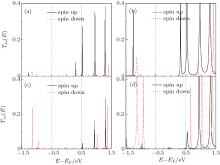Fig.  3. Spin-dependent transmission near the Fermi energy with different interfacial coupling strengths at 1.0  V and − 1.0  V: (a) β = 0.2, V = 1.0  V; (b) β = 0.6, V = 1.0  V; (c) β = 0.2, V = − 1.0  V; and (d) β = 0.6, V = − 1.0  V.

Figure  4(a) gives the dependence of the current on β at ± 1.0  V. A clear exponential relation is obtained for both the charge current and the spin current at ± 1.0  V with Ic(s) (V) ∝ eac(s)(Vβ . Obviously, the coefficient ac(s)(V) relies on the bias, which is much larger at the positive bias. It is because the tunneling barrier consists of the interfacial component and the central molecular one. The latter is related to the wave functions of the orbitals, which are modulated by the bias. In the following, we further calculate the dependence of the rectification ratio on the interfacial coupling strength at ± 1.0  V, where the charge-current rectification ratio and the spin-current rectification ratio are defined as RR(V) = − Ic(V)/Ic(− V) and SRR(V) = − Is(V)/Is(− V), respectively. Figure  4(b) displays a nonlinear dependence of the rectification ratio on β . The RR (SRR) increases quickly with β and approaches a maximum value of 21.1 (18.5) around β = 0.3, and then drops to 15.8 (14.8) at β = 1.2. The existence of an inflection point in the curve can be understood as follows. In the limitation of a weak or a strong interfacial coupling, the current will go to zero or extremely large at both positive and negative biases due to the exponential dependence. In either case, the rectification ratio should tend to be 1.0. As a result, an inflection point will appear at an intermediate value of β . This means that a moderate interfacial coupling is advantageous to realize a large rectification ratio, which may be adjusted in experiments by using a suitable anchoring group to contact the molecule with the electrodes.

 Figure OptionFig.  4. (a) Charge current and spin current at ± 1  V with different interfacial coupling parameters. (b) Dependence of charge current rectification ratio (RR) and spin current rectification ratio (SRR) at | V| = 1.0  V on the interfacial coupling parameter.

A lingering question is whether this inflection point is related to the structural asymmetry of the device. In the present device, the structural asymmetry is induced by the spin correlation, with radical spins in the left organic ferromagnetic part. In Fig.  5, the RR (SRR) dependence on β is calculated at two different values of J. With J = 1.2, the inflection point appears at β = 0.55 for RR and at β = 0.60 for SRR. With a larger J of 1.8, the inflection point becomes β = 1.2 for both RR and SRR. This means that the optimal coupling strength relies on the spin correlation parameter J. The inflection points for spin-current rectification and charge-current rectification may be different essentially.

 Figure Option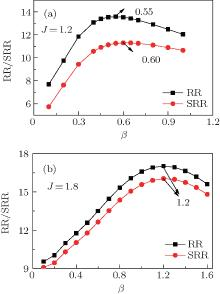Fig.  5. Dependence of RR and SRR at | V| = 1.0  V on the interfacial coupling parameter with (a) J = 1.2 and (b) J = 1.8.

3.2. Spin-resolved interfacial coupling

Now we start to discuss the spin resolved interfacial coupling effect on the rectification. Here the interfacial coupling parameters with different spins are distinguished as β u and β d. In Fig.  6, we first fix β u = 1.0 and calculate the dependence of rectification on β d. It is found that when β d changes from 0.3 to 1.2, the current– voltage curve is modified little both for charge current and spin current, which only happens under large positive biases. The rectification ratio at | V| = 1.0  V is calculated with the ratio of γ d/u = β d/β u. A slight increase of about 1.0 is observed for the RR, while the SRR decreases correspondingly.

 Figure Option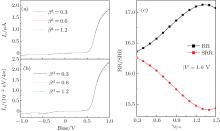Fig.  6. (a) Charge current and (b) spin current as a function of bias with different spin-down interfacial coupling parameters. Here the spin-up interfacial coupling is fixed with β u = 1.0. (c) RR and SRR at | V| = 1.0  V versus the ratio of β d/β u.

The dependence of the rectification ratio on γ d/u can be understood from the spin-dependent transmission spectrum. Figure  7 shows the transmission at γ d/u = 0.3 and 1.2. It can be found that the current at − 1.0  V is only contributed by the spin-up transmission. In the case of fixed β u, the spin-up transmission peak is undisturbed. Thus the current at negative bias remains unchanged. At + 1.0  V, besides the large spin-up LUMO transmission, a very weak transmission peak induced by the spin-down LUMO also appears in the bias window. When γ d/u increases from 0.3 to 1.2, the height of the spin-down LUMO peak is enhanced from a negligible quantity to a notable value (∼ 0.04). As a result, the current, as a sum of the spin-up and spin-down currents, increases slightly, with a similar dependence on the coupling strength as discussed above. However, as a difference of the spin-up and spin-down currents, the spin current decreases correspondingly.

 Figure Option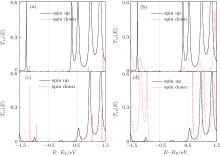Fig.  7. Spin-dependent transmission at ± 1.0 V with different spin-resolved interfacial coupling parameters: (a) β u = 1.0, β d = 0.3, V = 1.0  V; (b) β u = 1.0, β d = 1.2, V = 1.0  V; (c) β u = 1.0, β d = 0.3, V = − 1.0  V; and (d) β u = 1.0, β d = 1.2, V = − 1.0  V.

Due to the spin splitting in the central molecule, the variation of spin-up and spin-down interfacial coupling will be asymmetric for the transport. So, we further investigate another spin-revolved interfacial coupling by fixing β d. As shown in Fig.  8, with the increase of β u the current increases distinctly. Particularly, we notice that at β u = 0.3, the spin current does not follow the charge current under large positive biases, where its magnitude goes down to zero. The SRR in Fig.  8(c) is negative at β u = 0.3, which indicates that the spin orientation of the spin current at the positive bias is reversed and an antiparallel spin-current rectification occurs. Figure  8(c) shows a notable modulation of the rectification ratio by interfacial spin-splitting parameter γ u/d = β u/β d. When γ u/d decreases from 1.5 to 0.3, the RR is enhanced significantly from 14 to 31, while the SRR changes from 13 to − 5. The result means that in this case, a weak γ u/d or β u is advantageous to obtaining a large charge-current rectification. Vice versa, a strong γ u/d or β u is helpful to realizing a strong parallel spin-current rectification.

 Figure Option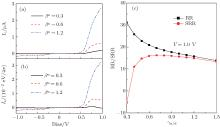Fig.  8. (a) Charge current and (b) spin current as a function of bias with different spin-up interfacial coupling parameters. Here the spin-down interfacial coupling is fixed, with β = 1.0. (c) RR and SRR at | V| = 1.0  V versus the ratio of β u/β d.

Such modulation of the rectification ratio can be deduced from the transmission shown in Fig.  7. Since the transport is dominated by the spin-up transmission, β u (γ u/d) can be roughly seen as the total interfacial coupling strength. As discussed above, the inflection point exists at about β = 0.3. Thus, when β u (γ u/d) decreases to 0.3, the RR should increase. However, the smaller β u (γ u/d) will suppress the spin-up current as well as the spin current at positive bias. So the SRR will decrease with β u (γ u/d) decreasing. When the spin-up transmission is lower than the spin-down one at β u = 0.3, the spin-polarized orientation of the spin current will be reversed. It can be concluded that the interfacial manipulation on the spin with an opposite spin orientation from the radical spin order, e.g., inserting a spin selective barrier at the interface, will generate a more effective effect on the spin diode.

Note that the asymmetric interfacial coupling is not included in the present work. An asymmetric interfacial coupling may work as another source of rectification, which will compete with the intrinsic structural asymmetric and complicate the rectification mechanism of the spin diode.[28, 29] Moreover, when it is extended to the asymmetric spin-resolved coupling, the situation will become much more complex with various possibilities. A vast calculation is necessary to clarify this issue, which will be performed in our further studies.

4. Summary

We have performed a theoretical study of the interfacial coupling effect in an organic magnetic co-oligomer diode by calculating the rectification property under spin-independent and spin-resolved interfacial coupling. In the spin-independent coupling, the rectification behavior is robust against the interfacial coupling strength, while the rectification ratio is modulated effectively. An optimal interfacial coupling strength is observed to realize the largest rectification, which relies on the structural asymmetry of the molecule. In the case of spin-resolved coupling, we found that the manipulation of spin-up interfacial coupling, whose spin orientation is opposite to the radical spins, is more effective on the rectification. A weak spin-up coupling is advantageous to realize a large charge-current rectification, while a strong one is good for the spin-current rectification. An interfacial coupling induced transition for the spin-current rectification is also observed, where the parallel spin-current rectification changes to an antiparallel one companied with the reversal of spin-polarized orientation. This work is helpful to explore the potential interfacial modulation on the rectification in magnetic co-oligomer diodes.

Reference
 1 Naber W J M, Faez S and van der Wiel W G 2007 J. Phys. D: Appl. Phys. 40 R205 DOI:10.1088/0022-3727/40/12/R01 [Cited within:1] 2 Dediu V A, Hueso L E, Bergenti I and Taliani C 2009 Nat. Mater. 8 707 DOI:10.1038/nmat2510 [Cited within:1] 3 Sugawara T and Matsushita M M 2009 J. Mater. Chem. 19 1738 DOI:10.1039/b818851n [Cited within:1] 4 Pramanik S, Stefanita C G, Patiband la S, Band yopadhyay S, Garre K, Harth N and Cahay M 2007 Nat. Nanotechnol. 2 216 DOI:10.1038/nnano.2007.64 [Cited within:1] 5 Korshak Y V, Medvedeva T V, Ovchinnikov A A and Spector V N 1987 Nature 326 370 DOI:10.1038/326370a0 [Cited within:1] 6 Cao Y, Wang P, Hu Z, Li S Z, Zhang L Y and Zhao J G 1988 Synth. Met. 27 B625 DOI:10.1016/0379-6779(88)90209-3 [Cited within:1] 7 Iwamura H, Sugawara T, Itoh K and Takui T 1985 Mol. Cryst. Liq. Cryst. 125 379 [Cited within:1] 8 Katulevskii Y A, Magrupov M A and Murninov A A 1991 Phys. Status Solidi A 127 223 DOI:10.1002/(ISSN)1521-396X [Cited within:1] 9 Manriquez J M, Yee G T, McLean R S, Epstein A J and Miller J S 1991 Science 252 1415 DOI:10.1126/science.252.5011.1415 [Cited within:1] 10 Raju N P, Savrin T, Prigodin V N, Pokhodnya K I, Miller J S and Epstein A J 2003 J. Appl. Phys. 93 6799 DOI:10.1063/1.1556120 [Cited within:1] 11 Sugano T, Blundell S J, Lancaster T, Pratt F L and Mori H 2010 Phys. Rev. B 82 180401 DOI:10.1103/PhysRevB.82.180401 [Cited within:1] 12 Yoo J W, Edelstein R S, Lincoln D M, Raju N P, Xia C, Pokhodya K I, Miller J S and Epstein A J 2006 Phys. Rev. Lett. 97 247205 DOI:10.1103/PhysRevLett.97.247205 [Cited within:1] 13 Li B, Zhou M, Lu Y, Kao C K, Yoo J W, Prigodin V N and Epstein A J 2012 Org. Electron. 13 1261 DOI:10.1016/j.orgel.2012.03.038 [Cited within:1] 14 Hu G C, Guo Y, Wei J H and Xie S J 2007 Phys. Rev. B 75 165321 DOI:10.1103/PhysRevB.75.165321 [Cited within:2] 15 Wang W Z 2006 Phys. Rev. B 73 235325 DOI:10.1103/PhysRevB.73.235325 [Cited within:1] 16 Dalgleish H and Kirczenow G 2006 Phys. Rev. B 73 235436 DOI:10.1103/PhysRevB.73.235436 [Cited within:2] 17 Hu G C, He K L, Xie S J and Saxena A 2008 J. Chem. Phys. 129 234708 DOI:10.1063/1.3041773 [Cited within:6] 18 Hu G C, Wang H and Ren J F 2011 Chin. Phys. B 20 077306 DOI:10.1088/1674-1056/20/7/077306 [Cited within:3] 19 Schmeltzer D, Saxena A, Bishop A R and Smith D L 2003 Phys. Rev. B 68 195317 DOI:10.1103/PhysRevB.68.195317 [Cited within:1] 20 Souza F M, Egues J C and Jauho A P 2007 Phys. Rev. B 75 165303 DOI:10.1103/PhysRevB.75.165303 [Cited within:1] 21 Guo Y, Chen X Y, Zhai F, Gu B L and Kawazoe Y 2002 Appl. Phys. Lett. 80 4591 DOI:10.1063/1.1485121 [Cited within:1] 22 Merchant C A and Marković N 2008 Phys. Rev. Lett. 100 156601 DOI:10.1103/PhysRevLett.100.156601 [Cited within:1] 23 Dalgleish H and Kirczenow G 2006 Phys. Rev. B 73 235436 DOI:10.1103/PhysRevB.73.235436 [Cited within:1] 24 Zuo M Y, Hu G C, Li Y, Ren J F and Wang C K 2014 Chin. Phys. B 23 087306 DOI:10.1088/1674-1056/23/8/087306 [Cited within:1] 25 Zhu L, Yao K L and Liu Z L 2012 Chem. Phys. 397 1 DOI:10.1016/j.chemphys.2011.09.009 [Cited within:1] 26 Yaliraki S N, Kemp M and Ratner Mark A 1999 J. Am. Chem. Soc. 121 3428 DOI:10.1021/ja982918k [Cited within:3] 27 Chen F, Li X L, Hihath J, Huang Z F and Tao N J 2006 J. Am. Chem. Soc. 128 15874 DOI:10.1021/ja065864k [Cited within:1] 28 Zhou C, Deshpand e M R, Ree d M A, Jones II L and Tour J M 1997 Appl. Phys. Lett. 71 611 DOI:10.1063/1.120195 [Cited within:2] 29 Jeremy T, Mads B and Kurt S 2002 Phys. Rev. Lett. 89 138301 DOI:10.1103/PhysRevLett.89.138301 [Cited within:2] 30 Nicolae A, Jens B, Lazić P, Caciuc V, Hoffmann G, Wiesendanger R and Blügel S 2010 Phys. Rev. Lett. 105 066601 DOI:10.1103/PhysRevLett.105.066601 [Cited within:1] 31 Sanvito S 2010 Nat. Phys. 6 562 DOI:10.1038/nphys1714 [Cited within:1] 32 Fang Z, Liu Z L and Yao K L 1994 Phys. Rev. B 49 3916 DOI:10.1103/PhysRevB.49.3916 [Cited within:4] 33 Hu G C, Wang H, Ren J F, Xie S J and Timm C 2014 Org. Electron. 15 118 DOI:10.1016/j.orgel.2013.10.028 [Cited within:3] 34 Datta S 1995 Electronic Transport in Mesoscopic Systems New York Oxford University Press 148 [Cited within:2] 35 Xie S J, Zhao J Q, Wei J H, Wang S G, Mei L M and Han S H 2000 Europhys. Lett. 50 635 DOI:10.1209/epl/i2000-00317-0 [Cited within:1] 36 Sayed S Y, Fereiro J A, Yan H, McCreery R L and Bergren A J 2012 Proc. Natl. Acad. Sci. USA 109 11498 DOI:10.1073/pnas.1201557109 [Cited within:1] 37 Yu Y J, Li Y Y, Wan L H, Wang B and Wei Y D 2013 Mod. Phys. Lett. B 27 1350121 [Cited within:1] 38 Hu G C, Wei J H and Xie S J 2007 Appl. Phys. Lett. 91 142115 DOI:10.1063/1.2790076 [Cited within:2] 39 Fan Z Q and Chen K Q 2010 Appl. Phys. Lett. 96 053509 DOI:10.1063/1.3309708 [Cited within:1] 40 Zeng J, Chen K Q, He J, Zhang X J and Sun C Q 2011 J. Phys. Chem. C 115 25072 DOI:10.1021/jp208248v [Cited within:1] 41 Hu G C, Zhang G P, Ren J F, Wang C K and Xie S J 2011 Appl. Phys. Lett. 99 082105 DOI:10.1063/1.3627169 [Cited within:1]